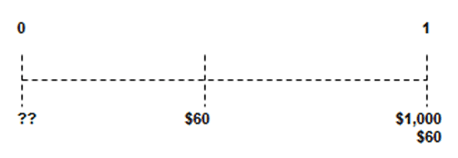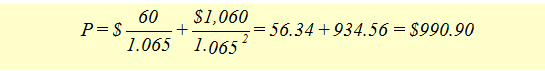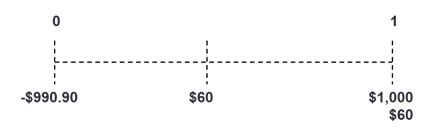Fixed Income

## Calculate the Value of a Coupon Paying Bond

The value of a coupon paying bond is calculated by discounting the future payments (coupon and principal) by an appropriate discount rate.

Suppose you have a bond with a $1,000 face value that matures 1 year from today. The coupon rate is 12% and the bond makes semi-annual coupon payments of$60. The bond yield is 13%. The cash flows from the bond are depicted below:The bond characteristics are summarized below:

• Par Value =     $1,000 • Yield = 13% annual (13/2 =6.5% semi-annual) • Coupon = 12% with semi-annual payment of$60
• Maturity   =      1 year

The value of the bond is calculated as follows:Note that the coupon is paid semi-annually, i.e., \$60 per 6 months. The discounting is also done semi-annually.The general bond pricing formula for all bonds can be stated as:Where:

• Pi \=  the price of the bond i
• Ct \= cash flow from the bond i at time t
• ri \= the annualized yield to maturity on bond i
• M = the time in years until the bond matures